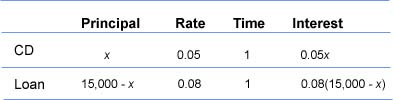Simple Interest and Money Problems

Simple Interest and Money Problems

One type of money problem involves simple interest. The simple interest formula is:

interest = principal  x  rate  x  time

When working with interest problems involving two amounts, we often let the variable represent one amount of money, then represent the second amount in terms of the first. Other types of money problems may include finding costs for sales, unit prices, amounts of product, or adjustments in inventory.

Example

Jose has \$15,000 to invest. He is considering two investments. One is a loan he can make to another party that pays him 8% simple interest for a year. A second investment is a 1-year certificate of deposit that pays 5%. Jose decides to place some money in each investment, and earns a total of \$1,125 interest in 1 year from the two investments. How much money does he spend on each investment?

Understand
Let x = amount to be invested at 5%.
Then 15,000 - x = amount to be invested at 8%.
The sum of the investments is \$1,125.

Use the simple interest formula to solve this problem.
interest = principal  x  rate  x  timeTranslate
(interest from 5% CD) + (interest from 8% investment) = total interest
0.05x             +        0.08(15,000 - x)           = 1,125

Solve
0.05x + 0.08(15,000 - x) = 1,125
0.05x + 0.08(15,000) - 0.08x = 1,125    distributive property
0.05x + 1200 - 0.08x = 1,125               multiply
-0.03x + 1200 = 1,125                        combine like terms
-0.03x = -75                                     subtract 1,200 from both sides
x = -75/-0.03 = 2,500                         divide both sides by -0.03